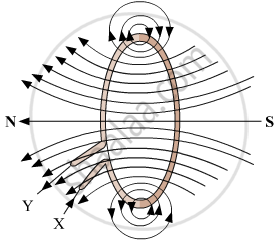# Answer the Following Question. Explain with the Help of the Pattern of Magnetic Field Lines the Distribution of the Magnetic Field Due to a Current-carrying a Circular Loop. - Science

Diagram
Short Note

Explain with the help of the pattern of magnetic field lines the distribution of the magnetic field due to a current-carrying a circular loop.

#### Solution

The distribution of the magnetic due to a circular current-carrying loop can be seen in the following diagram with the help of magnetic field lines:Concept: Magnetic Field Due to Current in a Loop (Or Circular Coil)
Is there an error in this question or solution?